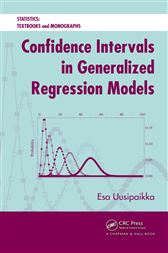Confidence Intervals in Generalized Regression Models

Series: Statistics: A Series of Textbooks and MonographsCA\$69.86
A Cohesive Approach to Regression Models

Confidence Intervals in Generalized Regression Models introduces a unified representation—the generalized regression model (GRM)—of various types of regression models. It also uses a likelihood-based approach for performing statistical inference from statistical evidence consisting of data and its statistical model.

Provides a Large Collection of Models

The book encompasses a number of different regression models, from very simple to more complex ones. It covers the general linear model (GLM), nonlinear regression model, generalized linear model (GLIM), logistic regression model, Poisson regression model, multinomial regression model, and Cox regression model. The author also explains methods of constructing confidence regions, profile likelihood-based confidence intervals, and likelihood ratio tests.

Uses Statistical Inference Package to Make Inferences on Real-Valued Parameter Functions

Offering software that helps with statistical analyses, this book focuses on producing statistical inferences for data modeled by GRMs. It contains numerical and graphical results while providing the code online.